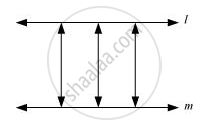# Give a Definition for Parallel Lines. Are There Other Terms that Need to Be Defined First? What Are They, and How Might You Define Them? - Mathematics

Give a definition for parallel lines. Are there other terms that need to be defined first? What are they, and how might you define them?

#### Solution

Parallel Lines :-

If the perpendicular distance between two lines is always constant, then these are called parallel lines. In other words, the lines which never intersect each other are called parallel lines.

To define parallel lines, we must know about point, lines, and distance between the lines and the point of intersection.Concept: Euclid’S Definitions, Axioms and Postulates
Is there an error in this question or solution?

#### APPEARS IN

NCERT Class 9 Maths
Chapter 5 Introduction to Euclid's Geometry
Exercise 5.1 | Q 2.1 | Page 85